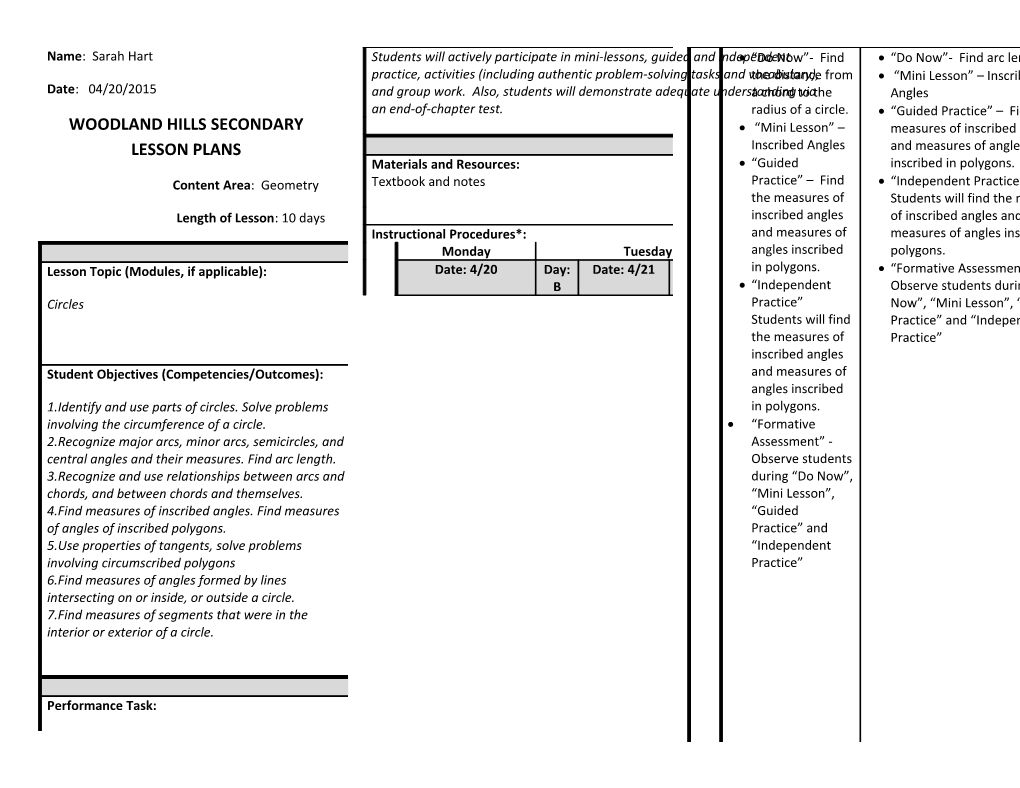# Name: Sarah HartName: Sarah Hart

Date: 04/20/2015

WOODLAND HILLS SECONDARY
LESSON PLANS

Content Area: Geometry

Length of Lesson:10 days

STAGE I – DESIRED RESULTS
Lesson Topic (Modules, if applicable):
Circles / Big Ideas:
There are some mathematical relationships that are always true and these relationships are used as the rules of arithmetic and algebra and are useful for writing equivalent forms of expressions and solving equations and inequalities.
Numbers, measures, expressions, equations, and inequalities can represent mathematical situations and structures in many equivalent forms. / Understanding Goals (Concepts):
Find angle measures of central, inscribed, interior, and exterior angles; find arc measure and length of circles. Special properties of circles are explored including the fact that a circle is a unique geometric shape in which the angles, arcs, and segments intersecting that circle have special relationships.
Student Objectives (Competencies/Outcomes):
1.Identify and use parts of circles. Solve problems involving the circumference of a circle.
2.Recognize major arcs, minor arcs, semicircles, and central angles and their measures. Find arc length.
3.Recognize and use relationships between arcs and chords, and between chords and themselves.
4.Find measures of inscribed angles. Find measures of angles of inscribed polygons.
5.Use properties of tangents, solve problems involving circumscribed polygons
6.Find measures of angles formed by lines intersecting on or inside, or outside a circle.
7.Find measures of segments that were in the interior or exterior of a circle. / Essential Questions:
How can you use coordinates and algebraic techniques to represent, interpret, and verify geometric relationships?
How can a change in one measurement of a 2- or 3-dimensional figure effect other measurements such as perimeter, area, surface area or volume of that figure? / Vocabulary:
Chord, circumference, arc, tangent, secant, center, central angle, circumscribed, diameter, inscribed, intercepted, major and minor arcs, point of tangency
STAGE II – ASSESSMENT EVIDENCE
Students will actively participate in mini-lessons, guided and independent practice, activities (including authentic problem-solving tasks and vocabulary), and group work. Also, students will demonstrate adequate understanding via an end-of-chapter test. / Formative Assessments:
Pre-assessments, open-ended higher-order-thinking questions, think-pair-share, graphic organizers, do nows, observation of guided and independent practice, brief in-class writing prompts
STAGE III – LEARNING PLAN
Materials and Resources:
Textbook and notes / Interventions:
Flexible grouping, students will be encouraged to attend Math Lab and College and Career Access Center tutoring.
Instructional Procedures*:
Monday / Tuesday / Wednesday / Thursday / Friday
Date: 4/20 / Day: B / Date: 4/21 / Day: A / Date: 4/22 / Day: B / Date: 4/23 / Day: A / Date: 4/24 / Day: B
Procedures /
• “Do Now”- Find the distance from a chord to the radius of a circle.
• “Mini Lesson” – Inscribed Angles
• “Guided Practice” – Find the measures of inscribed angles and measures of angles inscribed in polygons.
• “Independent Practice”
Students will find the measures of inscribed angles and measures of angles inscribed in polygons.
• “Formative Assessment” - Observe students during “Do Now”, “Mini Lesson”, “Guided Practice” and “Independent Practice”
/
• “Do Now”- Find arc length
• “Mini Lesson” – Inscribed Angles
• “Guided Practice” – Find the measures of inscribed angles and measures of angles inscribed in polygons.
• “Independent Practice”
Students will find the measures of inscribed angles and measures of angles inscribed in polygons.
• “Formative Assessment” - Observe students during “Do Now”, “Mini Lesson”, “Guided Practice” and “Independent Practice”
/
• “Do Now”- Write three lines on “tangent”
• “Mini Lesson” –Tangents
• “Guided Practice” – Use properties of tangents; solve problems involving circumscribed polygons.
• “Independent Practice” – Students will use properties of tangents and solve problems involving circumscribed polygons.
• “Formative Assessment” - Observe students during “Do Now”, “Mini Lesson”, “Guided Practice” and “Independent Practice”
/
• “Do Now”- Find the measure of a tangent.
• “Mini Lesson” –Tangents
• “Guided Practice” – Use properties of tangents; solve problems involving circumscribed polygons.
• “Independent Practice” – Students will use properties of tangents and solve problems involving circumscribed polygons.
“Formative Assessment” - Observe students during “Do Now”, “Mini Lesson”, “Guided Practice” and “Independent Practice” /
• “Do Now”- Write five lines about what you know about circles. Underline at least 3 vocabulary words.
• “Mini Lesson” –Secants, Tangents, and Angle Measures
• “Guided Practice” – Find measures of angles formed by lines intersecting on or inside, or outside a circle. Find measures of segments that were in the interior or exterior of a circle
• “Independent Practice”
Students will find measures of angles formed by lines intersecting on, inside, or outside a circle and find measures of segments that were in the interior or exteriori of a circle.
• “Formative Assessment” - Observe students during “Independent Practice”

Assignments / 10.4 Day 1
Pages 549-550 8-10, 13-16, 22, 25 / 10.4 Day 2 Worksheet / 10.5 Day 1 Pages 556-557 8-18, 23 / 10.5 Day 2 Worksheet / 10.6 Day 1
Pages 564-565 12-32 even

*Include Do Now, Mini Lesson, Guided Practice, Independent Practice, Summations/Formative Assessments, Reflections